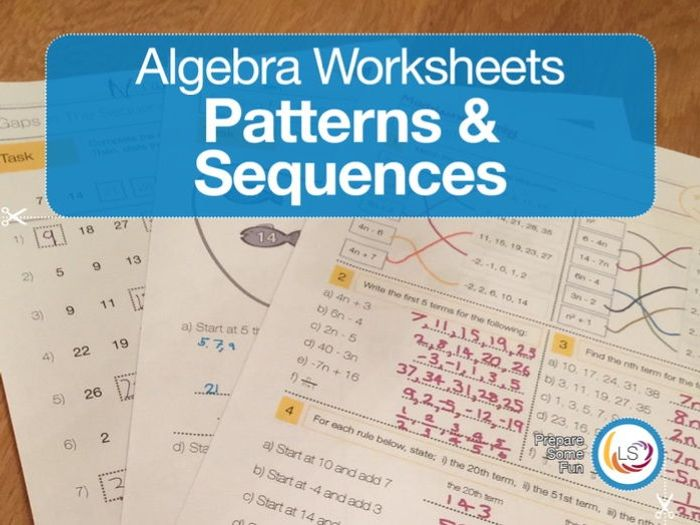This pack is filled with worksheet activities covering the topic of patterns and sequences. All worksheets are differentiated, offering supported worksheets, as well as worksheets which challenge the most able students. Answer sheets are included.

Gaps In The Sequence asks students to find the missing numbers of sequences (some linear, some following patterns such as doubling each number) and to state the term to term rule.

The Fencing In and Dinner Table Conundrum worksheets introduce the idea of geometric patterns which grow at a linear rate, giving a visual representation on linear sequences. For the more able students, this worksheet asks them to find the nth term of the given patterns.

Fishing for Sequences asks students to find numbers which appear in a given sequence. The first differentiation covers only term to term rules. The middle differentiation includes nth term examples. The most challenging differentiation asks students to state with algebraic reasoning, why a given number is not in a sequence. This checks for a true understanding of sequences.

The fifth worksheet is all about the nth term, with a more challenging worksheet that pushes students to think about such sequences as square numbers, and fraction sequences. The sixth worksheet continues the nth term exploration, starting with students identifying whether a given sequences is linear, and stating whether a given number is in a sequence.

The seventh worksheet, Function Machines, asks students to find the given rule for some numbers. And finally, worksheet eight offers a unique challenge for uniquely able students. This worksheet introduces students to the proper mathematical notation for linear sequences, including the formula for finding any term in a sequence.

You may also like:
Solving Linear Equations Follow Me Cards
Understanding and Manipulating Algebra Worksheets
\$5.06
Save for later

### Info

Created: Dec 29, 2015

Updated: Feb 22, 2018

pdf, 2 MB

MATW_ALG_ALG01A

jpg, 107 KB

StandardPreview

Report a problem